# MCQs on Control Systems

##### Page 22 of 23. Go to page 1 2 3 4 5 6 7 8 9 10 11 12 13 14 15 16 17 18 19 20 21 22 23
01․ When the system gain is doubled the gain margin becomes?
2 times
1/2 times
Remains unaffected
None of the above

Gain margin is the allowable gain. |G(jω)H(jω)| at phase crossover frequency = X Gain margin = 1/X Gain margin in db = 20 log(1/X) Gain margin ∝ 1/k Where, k = System gain. Therefore, if the system gain is doubled, gain margin is half i.e 1/2 times.

02․ A open loop transfer function is given as G(s) = (s+2)/(s+1)(s-1). Find the number of encirclements about '-1+j0'?
1
0
2
3

Characteristic equation is 1 + G(s) = 0 s²-1+s+2 = 0 s² + s + 1 = 0 Routh array table: s² --------- 1 1 s --------- 1 0 s0 --------- 1 There are no sign changes in first column of Routh array. Therefore, there are no closed loop poles in right half of s-plane. Number of encirclements N = P - Z Where P = Number of open loop poles in RHS of s-plane Z = Number of closed loop poles in RHS of s-plane Therefore, N = 1 - 0 = 1

03․ Find the stability for the following transfer function G(s) = 50/s(s+5) ?
Unstable
Marginally stable
Stable
Critically stable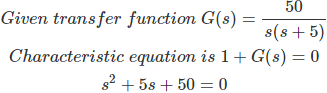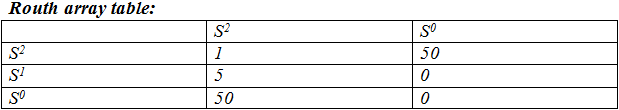There are no sign changes in first column of Routh array. Therefore, the system is stable.

04․ Find the corner frequency for the following transfer function? G(s) = 1/(s+2)

Corner frequency for first order function ωcf = 1/T Given function G(s) = 1/(s+2) G(s) = 1/2(1+s/2) G(s) = 1/(1+Ts) Therefore, T = 1/2 Corner frequency ωcf = 2 rad/s

05․ Error at corner frequency ωcf of first order control system is -------
±1 db
±2 db
±3 db
±4 db

Magnitude for first order factor is |F(jω)| = ±20 log √(1+(ωT)²) At corner frequency ω = ωcf = 1/T |F(jω)| = ±20 log √2 |F(jω)| = ± 3db

06․ The maximum phase lag occurs at the ------------------- of the two corner frequencies?
arithmetic mean
geometric mean
either 1 or 2
none of the above

Characteristics of phase lag compensator:
1. It shifts the gain crossover frequency to lower values where the desired phase margin is acceptable. Hence it is effective when the slope of the uncompensated system near the gain crossover frequency is high.
2. The maximum phase lag occurs at the geometric mean of the two corner frequencies.

07․ If the transfer function of a phase lead compensator is (s+a)/(s+b) and that of a lag compensator is (s+p)/(s+q), then which one of the following sets of conditions must be satisfied?
a > b, p > q
a > b, p < q
a < b, p < q
a < b, p >q

The transfer function of phase lead compensator = α(1 + TS)/(1 + αTS) Where α < 1 Therefore for lead compensator zero is nearer to origin. The transfer function of phase lag compensator = (1 + TS)/(1 + βTS) Where β > 1 Therefore for lag compensator pole is nearer to origin. Therefore, a < b, p >q

08․ The compensator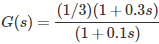would provide a maximum phase shift of
20o.
45o.
30o.
60o.

Given transfer function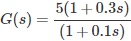The transfer function of phase lead compensator =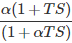By comparison, T = 0.3 α = 1/3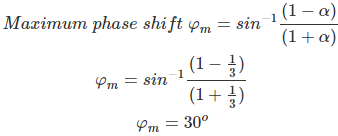09․ The transfer function of phase lead compensator is given by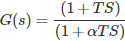, where, T > 0, α < 0. What is the maximum phase shift provided by this compensator?
sin-1((1 - α)/(1 + α)
cos-1((1 - α)/(1 + α)
tan-1((1 - α)/(2√α)
both 1 and 3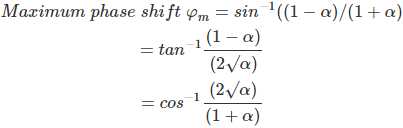10․ Consider the following statements for phase lead compensation:
Phase lead compensation shifts the gain crossover frequency to the right
The maximum phase lag occurs at the arithmetic mean of the two corner frequencies.
both 1 and 2
nether 1 nor 2

Characteristics of phase lag compensator:
1. It shifts the gain crossover frequency to lower values where the desired phase margin is acceptable. Hence it is effective when the slope of the uncompensated system near the gain crossover frequency is high.
2. The maximum phase lag occurs at the geometric mean of the two corner frequencies.

<<<20212223>>>